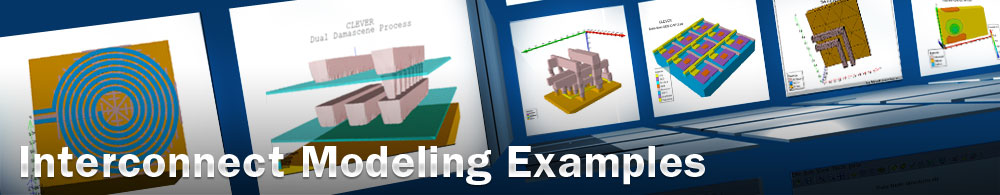Comparing one turn and straight line inductors

## questex11.in : Comparing one turn and straight line inductors

Requires: QUEST Minimum Versions: QUEST 2.0.2.R

This example demonstrates the advantages of straight line inductors.

Introduction:

It is often perceived that the highest inductance and Q factor are obtained using spiral inductors. This, however, is only true if the inductor has more than approximately 1.5 turns. Designers will often design 1 turn or 1.25 turn inductors out of habit, not realizing that a significantly better performing inductor can be designed with a straight line inductor.

The reason a straight inductor outperforms a one turn inductor in terms of inductance per length and Q factor is two fold:

1) If an inductor only has one turn, there is no added inductance from the mutual inductance of the other coils as occurs in a multi-turn inductor.

2) In a one turn inductor, inductance is lost due to the current travelling in opposite directions in the opposite conductor which is not mitigated by mutual inductance from other windings.

In a straight line inductor, there is also no mutual inductance benefit, BUT there is also no inductance lost due to opposite conductors having current flowing in opposite directions, because there are no opposite conductors in a straight line.

Experiment Description:

In this Quest experiment, two inductor layouts are compared:

(i) The first layout is a straight line inductor 480um long and 10um wide.

(ii) The second inductor has an identical length and width but is as a one turn design.

Results:

The results show that not only does the straight line inductor exhibit a greater inductance than its one turn counter part, but the maximum Q factor is also significantly higher.

Running the Example

To load and run this example, select the Load example button in DeckBuild. This will copy the input file, GDSII file and results to your current working directory. Select the run button to execute the example.

questex11_command.in is the quest input file common to the line and one turn inductor gds. Two simulations will thus be run one after the other.